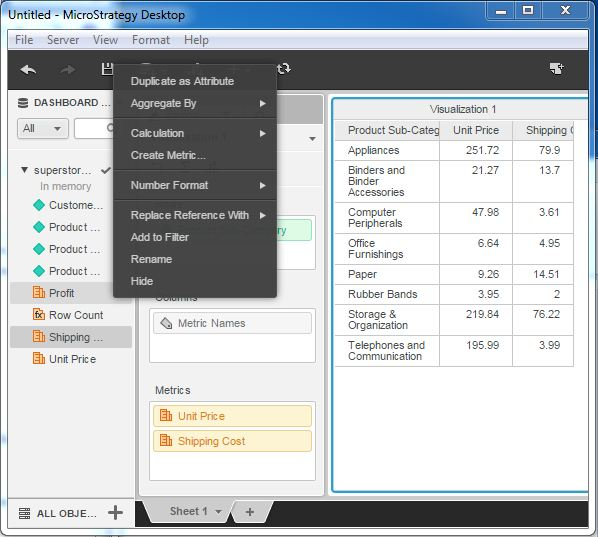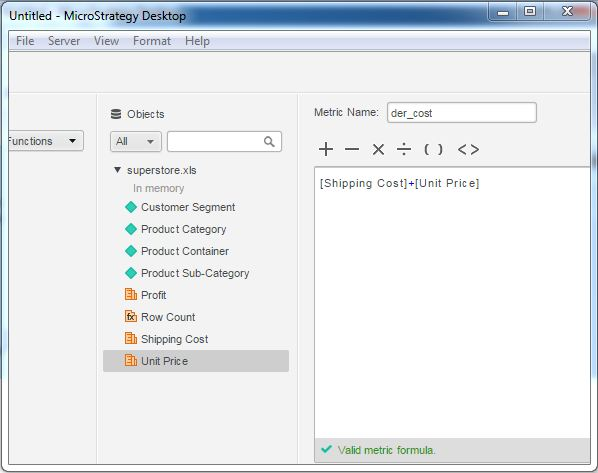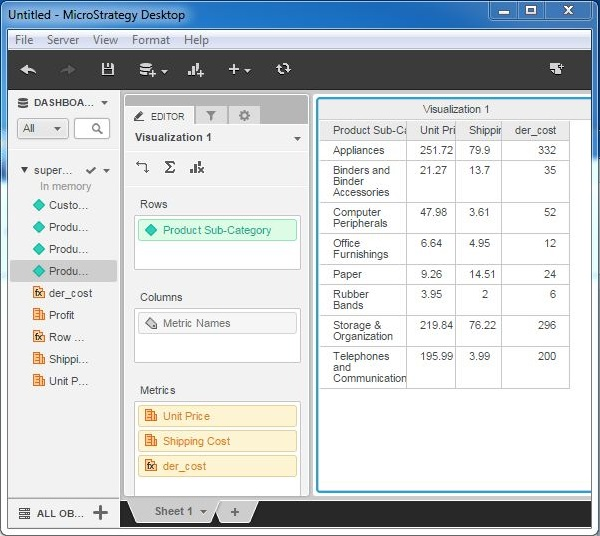# MicroStrategy - Creating Derived Metrics

Many times we need calculated metrics which are not already available in the data source. If such situations, metric values can be calculated from the existing metrics, using the create metric option. Thus, creating a derived metric is an approach to create values which we will need frequently in the report but which do not exist in the data source.

## Example

In this example, we are going to calculate the total of shipping cost and unit price for a product in the superstore sales data. Following are the steps to calculate it.

## Step 1

Let’s create a grip report using superstore sales. The report contains product-sub category as attribute and unit price as well as shipping cost as the metrics.## Step 2

Next, right-click near any of the metrics and choose the create metric option. It gives us a window to write the formula for the new metric. Here, write the formula we use in the existing metrics. The formula is as shown in the following screenshot.## Step 3

The new metric appears under the list of metric of the data source. We drag it to the existing grid report.